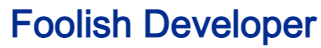Foolish Developer# Complete Guide about web designing - Foolish DeveloperThis tutorial will help you if you want to create a calculator using HTML and CSS. Here I have shared with you how to make a simple web calculator. Earlier I shared with you the design of many more types of calculators. However, it is very complex and very difficult for beginners to understand.

Here I have used HTML and CSS as well as the onclick function in HTML. If you know basic HTML and CSS, you can easily create them. With the help of this calculator, you can do simple addition, subtraction, multiplication, division calculations.

I created the buttons using HTML and then designed them with CSS. This calculator can only be created with the help of HTML. Here we have used CSS only for styling or design.

See the Pen
Untitled
by Foolish Developer (@foolishdevweb)
on CodePen.

Hopefully, the above live demo has helped you to know how this calculator works. Above you will find the required source code which you can copy and use in your own work.

## Simple Calculator using HTML CSS

To create this simple HTML calculator, first, create the HTML and CSS files. For this, you open any text editor or Notepad and create an HTML and CSS file. Then add those codes to the file. First, connect the HTML and CSS files to each other.

This calculator has been created using the following HTML codes. First I created the basic structure of a calculator. Then he made a display here in which the calculations can be seen.

Then we created another button that will help to delete all the information or clear the display. Then here I have made the necessary buttons with which I will do the calculation. Here 0 to 9 numbers and four operator buttons were created.

### HTML code of Simple Calculator

Every button here has been created with input and added the onclick function. When you click that button, the value that it contains will be displayed in the display. Copy all these codes and add them to your HTML file.

<div id=”calcContainer”>
<form name=”calculator”>
<!– top row –>
<div class=”result-clear”>
<!– Display –>

<!– Clear Button –>
<input type=”button” class=”clear” value=” AC ” onclick=”calculator.answer.value = ”” />
</div>
<!– All input button –>
<div class=”grid-buttons”>
<!– 2nd row –>
<input type=”button” value=”9″ onclick=”calculator.answer.value += ‘9’” />
<input type=”button” value=”8″ onclick=”calculator.answer.value += ‘8’” />
<input type=”button” value=”7″ onclick=”calculator.answer.value += ‘7’” />
<input type=”button” value=”+” onclick=”calculator.answer.value += ‘+'” class=”math” />
<br />
<!– 3rd row –>
<input type=”button” value=”4″ onclick=”calculator.answer.value += ‘4’” />
<input type=”button” value=”5″ onclick=”calculator.answer.value += ‘5’” />
<input type=”button” value=”6″ onclick=”calculator.answer.value += ‘6’” />
<input type=”button” value=”&minus;” onclick=”calculator.answer.value += ‘-‘” class=”math” />
<br />
<!– 4th row –>
<input type=”button” value=”1″ onclick=”calculator.answer.value += ‘1’” />
<input type=”button” value=”2″ onclick=”calculator.answer.value += ‘2’” />
<input type=”button” value=”3″ onclick=”calculator.answer.value += ‘3’” />
<input type=”button” value=”&#247;” onclick=”calculator.answer.value += ‘/'” class=”math” />
<br />
<!– 5th row –>
<input type=”button” value=”.” onclick=”calculator.answer.value += ‘.'” />
<input type=”button” value=”0″ onclick=”calculator.answer.value += ‘0’” />
<input type=”button” value=”&#215;” onclick=”calculator.answer.value += ‘*'” class=”math” />
</div>
</form>
</div>

### Design Basic Calculator with CSS

The following codes are the CSS codes that have been used to design this simple web calculator. First I did some basic design of the calculator. Then the display and the clear button have been designed. Then I designed each of the simple buttons and added a hover effect to each these buttons.

body {
background: #BDBDBD;
}
/* Basic Design */
#calcContainer {
width: 80%;
height: auto;
box-shadow: 0.5em 0.5em 1.2em rgba(0, 0, 0, 0.2);
position: absolute;
top: 50%;
left: 50%;
transform: translate(-50%,-50%);
background: #ffffff;
}
/* 1st row */
.result-clear {
display: flex;
margin: 0;
}
/* Display */
.result-clear input[type=text].result {
width: 72%;
height: 60px;
font-size: 1.4em;
font-family: ‘Roboto’, sans-serif;
display: block;
border: 0;
}
/* Input button */
.grid-buttons {
margin: 0;
justify-content: space-between;
align-items: center;
background: #212121;
display: flex;
flex-wrap: wrap;
}
input[type=button] {
font-family: ‘Roboto’, sans-serif;
font-size: 1.2em;
font-weight: 500;
color: #ffffff;
background: #212121;
border: 2px solid #212121;
flex-grow: 1;
width: 25%;
height: auto;
border: 0;
align-items: center;
text-align: center;
}
input[type=button]:focus {
outline: 0;
}
input[type=button].clear {
background: #009688;
border: 2px solid #009688;
width: 25.5%;
font-size: 20px;
}
.grid-buttons input[type=button].math {
background: #757575;
border: 2px solid #757575;
}
/* button Hover Effect */
.grid-buttons input[type=button]:hover,
input[type=button].clear:hover {
border: 2px solid #00BCD4;
transition: 0.3s ease;
background: #00BCD4;
}

Hopefully, you have been able to create this simple calculator using HTML and CSS above. If there is any problem while making a calculator with these codes, you can definitely let me know by commenting. Earlier I created many more types of calculators using HTML CSS and JavaScript.